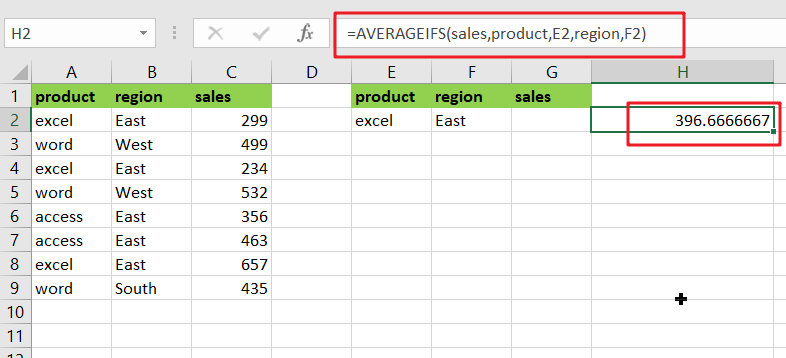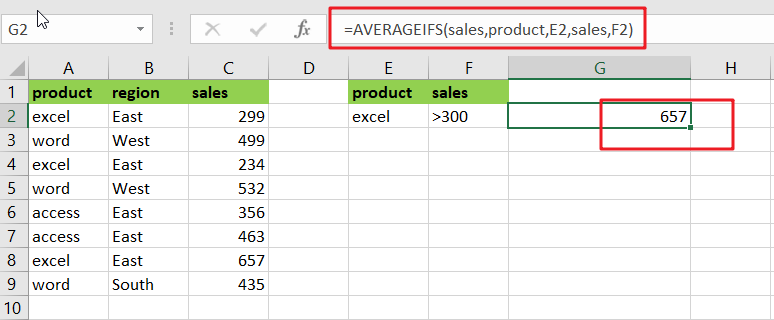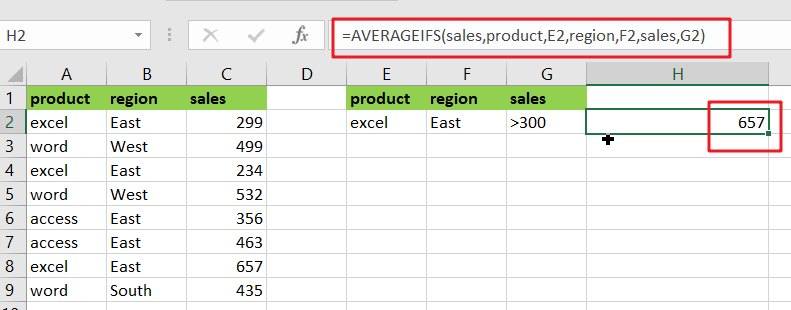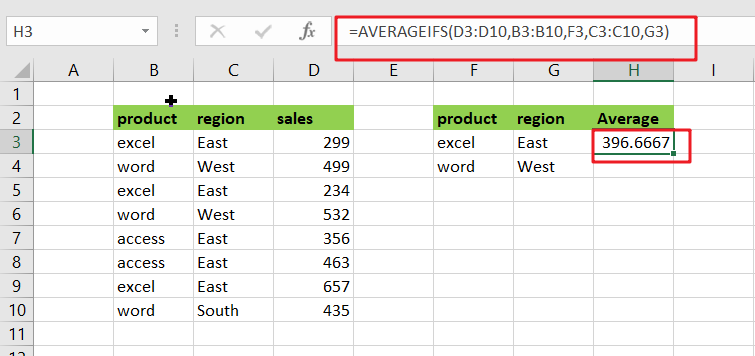# Average Of Numbers With Multiple Criteria In Excel

Have you ever come across a task to calculate the average of the numbers with respect to multiple criteria? Are you tired of doing this cumbersome task manually? Are you willing to do this task smartly in just a matter of seconds? Then congratulations because you have just landed on the right article.

In this article, you will get to know the easiest way to calculate the average of the numbers with respect to multiple criteria in no time.## General Formula

`=AVERAGEIFS(values,range1,criteria1,range2,criteria2)`

### Explanations for Syntax:

Before learning how to use the above formula, let’s first understand the role of each syntax in quickly calculating the average of the numbers concerning multiple criteria.

• `AVERAGEIFS`: In Excel, this function returns the average of a set of input values that meet multiple criteria. Learn more about the AVERAGEIFS Function.
• `Comma symbol` (,): It acts as a separator that helps to separate a list of values.
• `Parenthesis` (): The main function of this symbol is to group the elements.
• `Range`: It represents the input value from your worksheet.
• `Criteria`: The specific criteria or values used to calculate the average.

## Explanation

You can use the AVERAGEIFS function to average numbers based on multiple criteria. In the example, the formula in H2  is as follows:

`=AVERAGEIFS(sales,product,E2,region,F2)`Where “sales” (C2:C9), “product” (A2:A9), and “sales” (C2:C9) are named ranges.

The AVERAGEIFS function, like the COUNTIFS and SUMIFS functions, is intended to handle multiple criteria that are entered in [range, criteria] pairs. AVERAGEIFS’s behavior is fully automatic as long as this information is provided correctly.

In the example, we have product values in column E and region values in column F. We use these values directly by using cell references as criteria.

The first argument holds the range of values to average:sales range

To limit the calculation by product, we provide:product, E2

We use the following formula to limit the calculation by region:region, F2

The answer in cell H2 is 396:

Now, let’s go to the 2nd example in which we want to average the product of “excel” and the sales greater than 300. Please follow these instructions:

Enter the formula given below into a blank cell:

`=AVERAGEIFS(sales,product,E2,sales,F2)`(product range is the data that contains the criteria1, sales range is the range which we want to calculate the average, E2 and F2 are the criteria1 and criteria 2), then press the Enter key to get the desired result. Take a look at this screenshot:

Note: If you need more than two criteria, add the criteria ranges and criteria you require as follows:

`=AVERAGEIFS(sales,product,E2,region,F2,sales,G2)`Note: the first criteria range is product and E2, the second criteria range is region and F2 and the third criteria is sales and G2 and the range you want to average the values is the criteria, sales range.

For Better understanding, we are adding up the 3rd example as follows:

• Consider the example below to calculate the average of numbers based on multiple criteria.
• The image below will show the input values for Columns B, C, and D.
• Next, enter the given formula in the formula bar section.
• Finally, we’ll see the result in cell H3.## Summary

This article explains how to use the formulas in Excel to calculate the average of numbers with multiple criteria. I hope you found this article interesting. If you have any ideas, please share them with us.

### Related Functions

• Excel AVERAGEIFS function
The Excel AVERAGEAIFS function returns the average of all numbers in a range of cells that meet multiple criteria.The syntax of the AVERAGEIFS function is as below:= AVERAGEIFS (average_range, criteria_range1, criteria1, [criteria_range2, criteria2],…)….
• Excel COUNTIFS function
The Excel COUNTIFS function returns the count of cells in a range that meet one or more criteria. The syntax of the COUNTIFS function is as below:= COUNTIFS(criteria_range1, criteria1, [criteria_range2, criteria2]…)…
• Excel SUMIFS Function
The Excel SUMIFS function sum the numbers in the range of cells that meet a single or multiple criteria that you specify. The syntax of the SUMIFS function is as below:=SUMIFS (sum_range, criteria_range1, criteria1, [criteria_range2, criteria2], …)…
Related Posts

How to Sum if Equal to Many Items or A Range in Excel

If we want to sum numbers from a range with criteria, we often select SUMIF of SUMIFS functions as the first choice to create a formula. The criteria can be a number or an array or a collection of some ...

How to Sum if Equal to X or Y in Excel

In daily work, if we want to sum numbers from a range, and only sum the numbers which being equal to X or Y in the range, we can create a formula with Excel build-in functions to get the result. ...

How to Sum Last N Days in Excel

In our daily life, we may want to sum amounts or sales for a specific period, for example in last N days. Sum numbers in Excel is easy to run, we can apply SUM function. But if we want to ...

How to Sum if Contains an Asterisk

To add numbers together we need to apply SUM function. And if we want to add numbers based on some conditions, we can add criteria with the help of SUMIFS function, SUMIFS can filter data with multiple criteria effectively. If ...

How to Sum in Vertical Range

If we want to add numbers based on some conditions in Excel worksheet, we can add criteria with the help of SUMIFS function, SUMIFS can filter data with multiple criteria effectively. In this article, we will introduce you the method ...

How to Sum in Horizontal Range

To add numbers together we need to apply SUM function. But if we want to add numbers based on some conditions, we can add criteria with the help of SUMIFS function, SUMIFS can filter data with multiple criteria effectively. In ...

How to Sum with Criteria and Or Logic in Excel

To add numbers together we need to apply SUM function. And if we want to add numbers based on some conditions, we can add criteria with the help of SUMIFS function, SUMIFS can filter data with multiple criteria effectively. If ...

How to Subtotal Values for Groups and Only Keep One Subtotal for A Group in Column

Sometimes we may meet the case that subtotal values for different groups and only record a subtotal value for one group in a column, for other cells in this group, keep them blank. Today we will introduce you how can ...

How to Sum by Formula If Cells Are Not Blank in Criteria Range

Sometimes we may meet the cases that some blank cells exist in criteria range or sum range. In most situations we will ignore them, so we need to filter data by ‘not blank/not empty’. Today we will introduce you how ...

How to Sum by Formula if Cell Ends with in Excel

We have introduced the method of sum numbers based on criteria “sum if cell begins with” (you can refer to ‘How to Sum Data if Begins with in Excel’ on our website). In this article, we will show you the ...

Sidebar